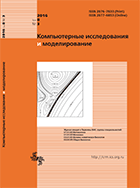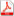Issue 3, 2016 Vol. 8

# All issues

Theoretical substantiation of the mathematical techniques for joint signal and noise estimation at rician data analysispdf (654K)  / List of references

The paper provides a solution of the two-parameter task of joint signal and noise estimation at data analysis within the conditions of the Rice distribution by the techniques of mathematical statistics: the maximum likelihood method and the variants of the method of moments. The considered variants of the method of moments include the following techniques: the joint signal and noise estimation on the basis of measuring the 2-nd and the 4-th moments (MM24) and on the basis of measuring the 1-st and the 2-nd moments (MM12). For each of the elaborated methods the explicit equations’ systems have been obtained for required parameters of the signal and noise. An important mathematical result of the investigation consists in the fact that the solution of the system of two nonlinear equations with two variables — the sought for signal and noise parameters — has been reduced to the solution of just one equation with one unknown quantity what is important from the view point of both the theoretical investigation of the proposed technique and its practical application, providing the possibility of essential decreasing the calculating resources required for the technique’s realization. The implemented theoretical analysis has resulted in an important practical conclusion: solving the two-parameter task does not lead to the increase of required numerical resources if compared with the one-parameter approximation. The task is meaningful for the purposes of the rician data processing, in particular — the image processing in the systems of magnetic-resonance visualization. The theoretical conclusions have been confirmed by the results of the numerical experiment.

Keywords: probability density function, Rice distribution, likelihood function, maximum likelihood method, method of moments, signal to noise ratio, noise dispersion
Citation in English: Yakovleva T.V. Theoretical substantiation of the mathematical techniques for joint signal and noise estimation at rician data analysis // Computer Research and Modeling, 2016, vol. 8, no. 3, pp. 445-473
Citation in English: Yakovleva T.V. Theoretical substantiation of the mathematical techniques for joint signal and noise estimation at rician data analysis // Computer Research and Modeling, 2016, vol. 8, no. 3, pp. 445-473
DOI: 10.20537/2076-7633-2016-8-3-445-473
• . Signal and noise calculation at Rician data analysis by means of combining maximum likelihood technique and method of moments. // Computer Research and Modeling. 2018. — V. 10, no. 4. — P. 511.
• . Signal and noise parameters determination at rician data analysis by method of moments of lower odd orders. // Computer Research and Modeling. 2017. — V. 9, no. 5. — P. 717.
Please note that citation information may be incomplete as it includes data from Crossref cited-by program partners only.
Views (last year): 2. Citations: 2 (RSCI).

Full-text version of the journal is also available on the web site of the scientific electronic library eLIBRARY.RU

The journal is included in the Russian Science Citation Index

The journal is included in the List of Russian peer-reviewed journals publishing the main research results of PhD and doctoral dissertations.

International Interdisciplinary Conference "Mathematics. Computing. Education"

The journal is included in the RSCI

Indexed in Scopus